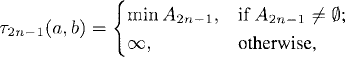# MARTINGALE CONVERGENCE THEOREMS

Martingale convergence theorems state that under certain conditions, a martingale, submartingale, or supermartingale converges to a limiting random variable. In this chapter, we shall present several martingale convergence theorems.

# 25.1 Basic Concepts and Facts

Definition 25.1 (Crossing of Real Numbers). Let {xn : n ≥ 0} be a sequence of real numbers. Let a < b be real numbers. The sequence {τn(a, b) : n ≥ 0} of crossings with respect to the sequence {xn : n ≥ 0} is defined by

(25.1a)and for every n ≥ 1, we have

(25.1b)(25.1c)where A2n−1 = {k ≥ τ2n−2 : xka} and A2n = {k ≥ τ2n−1 : xkb}.

Definition 25.2 (Crossing of Random Variables). Let{Xn : n ≥ 0} be a sequence of real random variables. Let a < b be real numbers. The sequence {τn(a, b) : n ≥ 0} of crossings with respect to the sequence {Xn : n ≥ 0} is defined as follows. For every ωΩ, {τn(a, b)(ω) : n ≥ 0} is the sequence of crossings with respect to the sequence {Xn(ω) : n ≥ 0}.

Definition 25.3 (). Let {n : n ≥ 0} be a filtration. Then is the σ-algebra generated by :

Theorem ...

Get Measure, Probability, and Mathematical Finance: A Problem-Oriented Approach now with the O’Reilly learning platform.

O’Reilly members experience live online training, plus books, videos, and digital content from nearly 200 publishers.